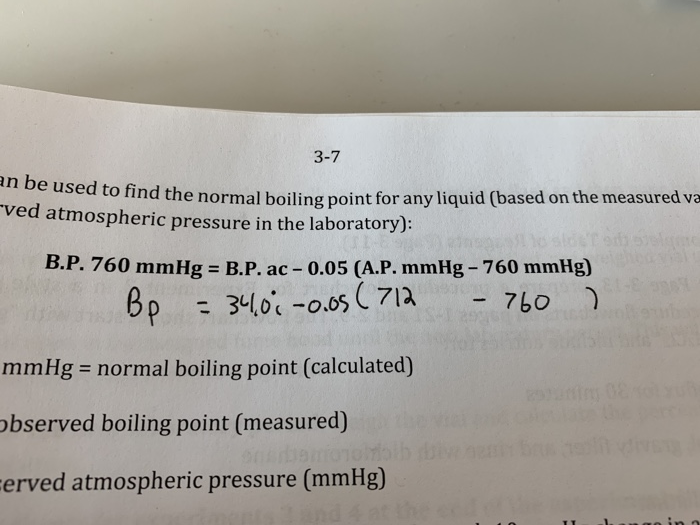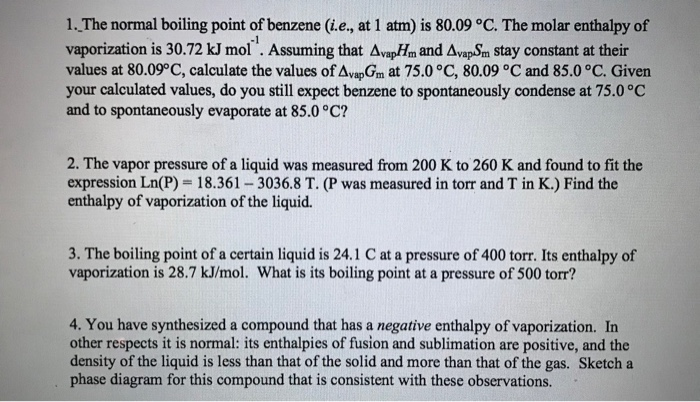Homework Help Question & Answers

# whats th B.P 760mmgh? 3-7 an be used to find the normal boiling po to find...whats th B.P 760mmgh?
3-7 an be used to find the normal boiling po to find the normal boiling point for any liquid (based on the measured va ved atmospheric pressure in the laboratory). B.P. 760 mmHg = B.P. ac -0.05 (A.P. mmHg - 760 mmHg) Bp = 34.00 -0.os (712 - 760 ) mmHg = normal boiling point (calculated) observed boiling point (measured) Ferved atmospheric pressure (mmHg)

#### Homework Answers

Answer #1

B.P. 760 mm Hg means boiling point of the liquid at a pressure of 760 mm Hg. 760 mm Hg is nothing but 1 atm. So, to evaluate boling point at atmospheric pressure, the given equation will be used.

Know the answer?
Your Answer:

#### Post as a guest

Your Name:

What's your source?

#### Earn Coin

Coins can be redeemed for fabulous gifts.

Not the answer you're looking for? Ask your own homework help question. Our experts will answer your question WITHIN MINUTES for Free.
Similar Homework Help Questions
• ### 1. The normal boiling point of benzene (i.e., at 1 atm) is 80.09 °C. The molar...1. The normal boiling point of benzene (i.e., at 1 atm) is 80.09 °C. The molar enthalpy of vaporization is 30.72 kJ mol . Assuming that AvapHm and AvapSm stay constant at their values at 80.09°C, calculate the values of AvapGm at 75.0 °C, 80.09 °C and 85.0 °C. Given your calculated values, do you still expect benzene to spontaneously condense at 75.0 °C and to spontaneously evaporate at 85.0°C? -1 2. The vapor pressure of a liquid was measured...

Free Homework App

Scan Your Homework
to Get Instant Free Answers
Need Online Homework Help?

Get Answers For Free
Most questions answered within 3 hours.